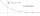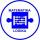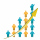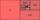# GP - sequence

The first three terms of a geometric sequence are as follows 10, 30. 90, find the next two terms of this sequence.

a4 =  270
a5 =  810
a6 =  2430

### Step-by-step explanation:Did you find an error or inaccuracy? Feel free to write us. Thank you!## Related math problems and questions:

• Sequences AP + GPThe three numbers that make up the arithmetic sequence have the sum of 30. If we subtract from the first 5, from the second 4 and keep the third, we get the geometric sequence. Find AP and GP members.
• Sum of GP membersDetermine the sum of the GP 30, 6, 1.2, to 5 terms. What is the sum of all terms (to infinity)?
• Six termsFind the first six terms of the sequence a1 = -3, an = 2 * an-1
• Geometric seqFind the third member of geometric progression if a1 + a2 = 36 and a1 + a3 = 90. Calculate its quotient.
• Geometric progressiobIf the sum of four consective terms of geometric progression is 80 and arithmetic mean of second and fourth term is 30 then find terms?
• Find next memberFind x if the numbers from a GP 7, 49, x.
• Find the 21Find the sum of the six terms of the finite geometric sequence 96, -48, 24, -12
• GP membersThe geometric sequence has 10 members. The last two members are 2 and -1. Which member is -1/16?
• AS - sequenceWhat are the first ten members of the sequence if a11=22, d=2.
• GP - three membersThe second and third of a geometric progression are 24 and 12(c+1), respectively, given that the sum of the first three terms of progression is 76. determine the value of c.
• AP - simpleFind the first ten members of the sequence if a11 = 132, d = 3.
• Geometric sequence 4It is given geometric sequence a3 = 7 and a12 = 3. Calculate s23 (= sum of the first 23 members of the sequence).
• Two geometric progressionsInsert several numbers between numbers 6 and 384 so that they form with the given GP numbers and that the following applies: a) the sum of all numbers is 510 And for another GP to apply: b) the sum of entered numbers is -132 (These are two different geome
• Missing term 2What is the missing term for the Geometric Progression (GP) 3, 15, 75,__, 1875?
• Three members GPThe sum of three numbers in GP (geometric progression) is 21 and the sum of their squares is 189. Find the numbers.
• Gp - 80One of the first four members of a geometric progression is 80. Find its if we know that the fourth member is nine times greater than the second.
• GP - 8 itemsDetermine the first eight members of a geometric progression if a9=512, q=2